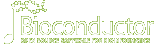# openPrimeRDOI: 10.18129/B9.bioc.openPrimeRThis is the development version of openPrimeR; for the stable release version, see openPrimeR.

## Multiplex PCR Primer Design and Analysis

Bioconductor version: Development (3.16)

An implementation of methods for designing, evaluating, and comparing primer sets for multiplex PCR. Primers are designed by solving a set cover problem such that the number of covered template sequences is maximized with the smallest possible set of primers. To guarantee that high-quality primers are generated, only primers fulfilling constraints on their physicochemical properties are selected. A Shiny app providing a user interface for the functionalities of this package is provided by the 'openPrimeRui' package.

Author: Matthias Döring [aut, cre], Nico Pfeifer [aut]

Maintainer: Matthias Döring <matthias-doering at gmx.de>

Citation (from within R, enter `citation("openPrimeR")`):

### Installation

To install this package, start R (version "4.2") and enter:

```if (!require("BiocManager", quietly = TRUE))
install.packages("BiocManager")

# The following initializes usage of Bioc devel
BiocManager::install(version='devel')

BiocManager::install("openPrimeR")```

For older versions of R, please refer to the appropriate Bioconductor release.

### Documentation

To view documentation for the version of this package installed in your system, start R and enter:

`browseVignettes("openPrimeR")`

 HTML R Script openPrimeR PDF Reference Manual Text NEWS

### Details

 biocViews Coverage, MultipleComparison, Software, Technology Version 1.19.0 In Bioconductor since BioC 3.6 (R-3.4) (5 years) License GPL-2 Depends R (>= 4.0.0) Imports Biostrings(>= 2.38.4), XML (>= 3.98-1.4), scales (>= 0.4.0), reshape2 (>= 1.4.1), seqinr (>= 3.3-3), IRanges(>= 2.4.8), GenomicRanges(>= 1.22.4), ggplot2 (>= 2.1.0), plyr (>= 1.8.4), dplyr (>= 0.5.0), stringdist (>= 0.9.4.1), stringr (>= 1.0.0), RColorBrewer (>= 1.1-2), DECIPHER(>= 1.16.1), lpSolveAPI (>= 5.5.2.0-17), digest (>= 0.6.9), Hmisc (>= 3.17-4), ape (>= 3.5), BiocGenerics(>= 0.16.1), S4Vectors(>= 0.8.11), foreach (>= 1.4.3), magrittr (>= 1.5), distr (>= 2.6), distrEx (>= 2.6), fitdistrplus (>= 1.0-7), uniqtag (>= 1.0), openxlsx (>= 4.0.17), grid (>= 3.1.0), grDevices (>= 3.1.0), stats (>= 3.1.0), utils (>= 3.1.0), methods (>= 3.1.0) LinkingTo Suggests testthat (>= 1.0.2), knitr (>= 1.13), rmarkdown (>= 1.0), devtools (>= 1.12.0), doParallel (>= 1.0.10), pander (>= 0.6.0), learnr (>= 0.9) SystemRequirements MAFFT (>= 7.305), OligoArrayAux (>= 3.8), ViennaRNA (>= 2.4.1), MELTING (>= 5.1.1), Pandoc (>= 1.12.3) Enhances URL Depends On Me openPrimeRui Imports Me Suggests Me Links To Me Build Report

### Package Archives

Follow Installation instructions to use this package in your R session.

 Source Package openPrimeR_1.19.0.tar.gz Windows Binary openPrimeR_1.19.0.zip (64-bit only) macOS Binary (x86_64) openPrimeR_1.19.0.tgz macOS Binary (arm64) openPrimeR_1.19.0.tgz Source Repository git clone https://git.bioconductor.org/packages/openPrimeR Source Repository (Developer Access) git clone git@git.bioconductor.org:packages/openPrimeR Package Short Url https://bioconductor.org/packages/openPrimeR/ Package Downloads Report Download StatsDocumentation »

Bioconductor

R / CRAN packages and documentationPlease read the posting guide. Post questions about Bioconductor to one of the following locations: